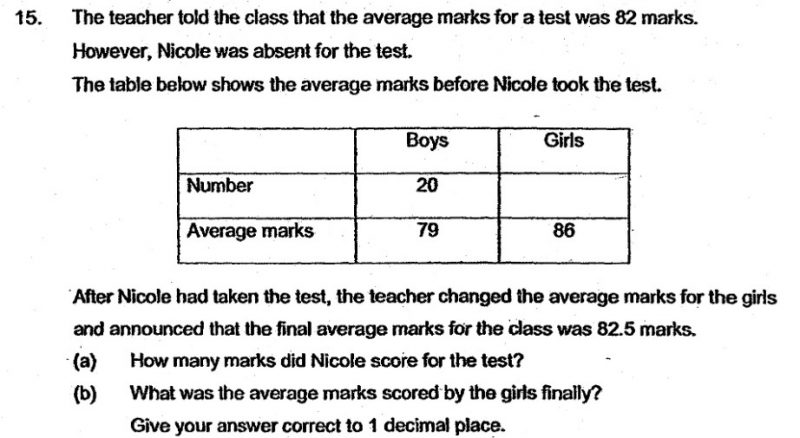# QuestionHi Parents,

Regards,

DNLNMUM

82 – 79 = 3

Each of the 20 boys need a contribution of 3 marks.

(20 x3)/(86 – 82)   = 60/4 = 15

There are 15 girls in the class.

82.5 – 82 = 0.5

The average score of the class must improve by 0.5 marks.

(a)            82 + 35 x 0.5 = 99.5

Nicole scored 99.5 marks.

(b)  Total Score of 15 girls = (82.5 ´ x 35)  – 20 x´ 79  =  1307.5

Score of Nicole = 1307.5 ÷ 15 = 87.2 1 d.p.